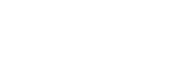# Bray Curtis Dissimilarity

The Bray Curtis dissimilarity is used to quantify the differences in species populations between two different sites. It’s used primarily in ecology and biology, and can be calculated with the following formula:Where:

• i & j are the two sites,
• Si is the total number of specimens counted on site i,
• Sj is the total number of specimens counted on site j,
• Cij is the sum of only the lesser counts for each species found in both sites.

## Simple Example

For a simple example, consider two aquariums;

• Tank one: 6 goldfish, 7 guppies and 4 rainbow fish,
• Tank two: 10 goldfish and 6 rainbow fish.

To calculate Bray-Curtis, let’s first calculate Cij (the sum of only the lesser counts for each species found in both sites). Goldfish are found on both sites; the lesser count is 6. Guppies are only on one site, so they can’t be added in here. Rainbow fish, though, are on both, and the lesser count is 4.
So Cij = 6 + 4 = 10.

Si ( total number of specimens counted on site i) = 6 + 7 + 4 = 17, and
Sj (total number of specimens counted on site j) = 10 + 6 = 16.

So our BCij = 1 – (2 * 10) / (17 + 16), or 0.39.

## Properties of the Bray Curtis Dissimilarity

The Bray-Curtis dissimilarity is always a number between 0 and 1. If 0, the two sites share all the same species; if 1, they don’t share any species.

To make it easy to work with, it’s often multiplied by 100, and then treated as a percentage. You may see a Bray Curtis dissimilarity of 0.21, for instance, being referred to as a Bray Curtis dissimilarity percent of 21%.

There’s another percentage which is often used to describe species counts; this one, though, tells you how similar two sites are rather than how different. It’s called the Bray Curtis index, and to calculate it you simply subtract the Bray Curtis dissimilarity (remember, a number between 0 and 1) from 1, then multiply by 100.

Let’s calculate this number for the fish example. The Bray Curtis dissimilarity was 0.39, and if we wanted it in terms of percentages we would have called it 39%. But the Bray Curtis index will be (1 – 0.39) · 100, or 61%. Notice this is, in a way, the opposite of the Bray Curtis dissimilarity. Identical sites have a Bray Curtis dissimilarity of 0, or 0%, and a Bray Curtis index of 100%. Sites which share no species would have a Bray Curtis dissimilarity of 1 (100%), and a Bray Curtis index of 0.

## Assumptions Used in Calculating the Bray-Curtis Dissimilarity

To calculate the Bray-Curtis dissimilarity between two sites you must assume that both sites are the same size, either in area or volume (as is relevant to species counts). This is because the equation doesn’t include any notion of space; it works only with the counts themselves.

If the two sites are not the same size, you will need to adjust your counts before doing the Bray-Curtis calculation.

## References

Measures of Distance Between Samples: Non-Euclidean
Retrieved from http://84.89.132.1/~michael/stanford/maeb5.pdf on March 9, 2018

Vavrek, Matthew. Tools for Paleontology: Distance/Similarity/Beta Diversity Indices
Retrieved from https://www.valencia.edu/~pardomv/pe/2011_1/238/distance.htm on March 9, 2018

CITE THIS AS:
Stephanie Glen. "Bray Curtis Dissimilarity" From StatisticsHowTo.com: Elementary Statistics for the rest of us! https://www.statisticshowto.com/bray-curtis-dissimilarity/
---------------------------------------------------------------------------Need help with a homework or test question? With Chegg Study, you can get step-by-step solutions to your questions from an expert in the field. Your first 30 minutes with a Chegg tutor is free!